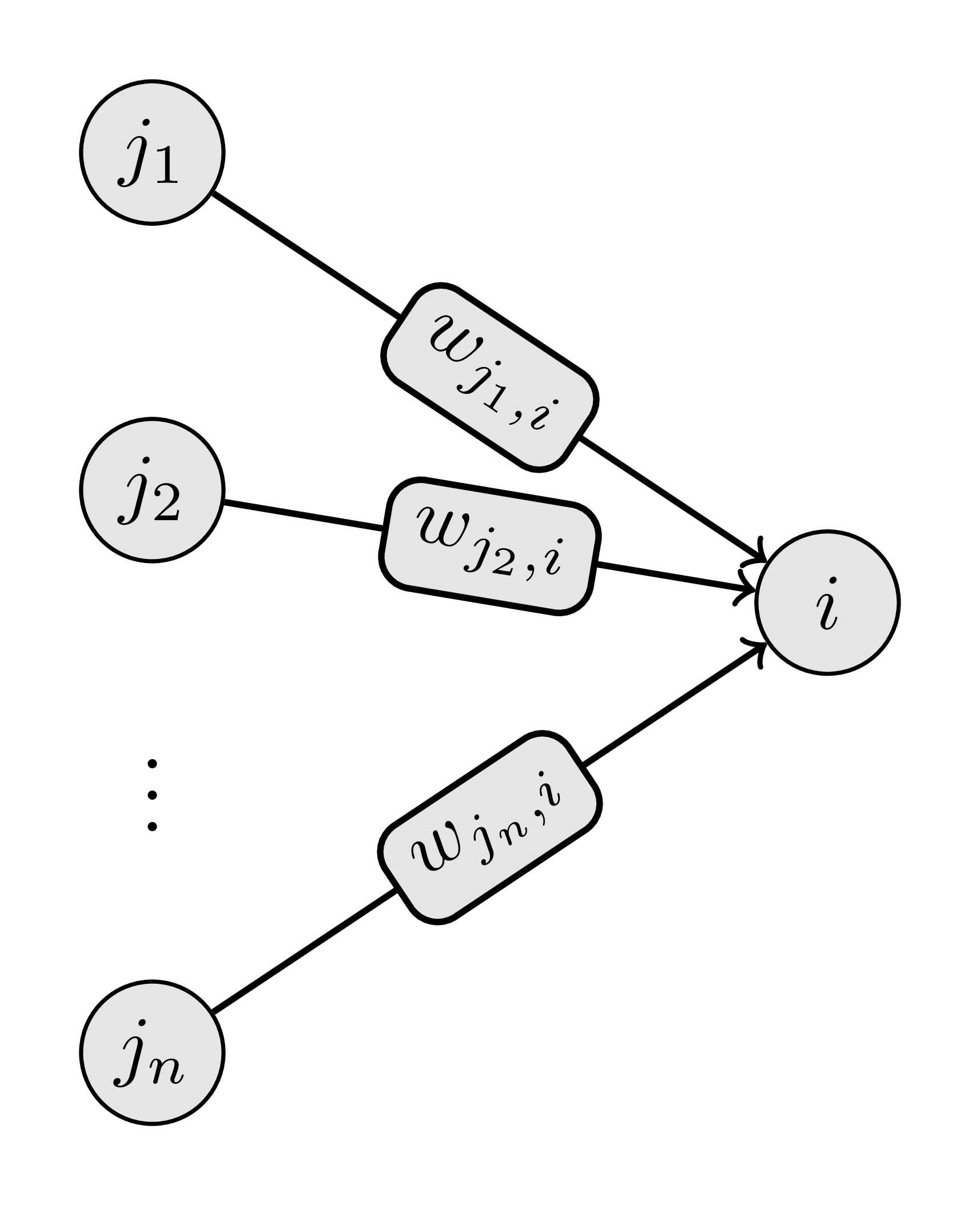# Neural Network¶

Pyrosim currently only uses an artificial neural networks to control the robots. This page is meant to be less of a tutorial on ANNs and more about pyrosim’s specific implementation of ANNs. However, there should be enough information to supply a basic understanding of how simple ANNs function. There are many resources for learning more about neural networks and Christopher Olah's blog is one of the prettier ones.

## Basics¶

ANNs are directed networks where the nodes are called neurons and the edges are called synapses (like a brain). They have many shapes and forms but will generally consist of some variation of input, output, and hidden neurons.

Input neurons receive their node value from some pre-specified method (like sensory input). The node values of the rest of the neurons are then updated based on the synaptic values coming from all of the input synapses. Synaptic values are calculated by multiplying the synaptic weight from the source neuron to the target neuron by the node value of the source neuron. These values are then computed using the activation function contained within the neuron. Most normally this is a summation of all the synaptic weight of all the inputs and a squashing function to keep the value within some range. The target neuron now contains its updated value and the rest of the network is updated in this same manner. After the network has been updated, node values are then generally read from the output neurons, giving the final result.

There are six built-in types of neurons in pyrosim

1. Sensor
2. Motor
3. Hidden
4. Bias
5. Function
6. User input

The ANNs used for robotic control tend not to have the strict input output neurons as in many networks used for machine learning. In pyrosim, the sensor, bias, function, and user input neurons are all types of input neurons which receive their values from different sources.

### Sensor Neurons¶

Sensor neurons are updated based on the value of the specified sensor and svi. This value is inputed to the network raw, meaning no thresholding will occur.

For example, if we want to input the distance from a ray sensor and the ray sensor has the id ray_sensor_handle we can use:

dist_neuron = sim.send_sensor_neuron(ray_sensor_handle, svi=0)


This will send a neuron to the simulation which, at every time step, reads the value from the ray sensor specified by by ray_sensor_handle at svi=0 (which is the distance component of the ray sensor) and assumes that value.

### Other Input Neurons¶

Bias, function, and user input neurons are the other types of input neurons. Unlike the sensor neuron They recieve their input from outside the simulation. Bias neurons maintain a constant value of 1. Function neurons get their value from a user-specified function mapped over the appropriate eval_time and dt. Finally, user input neurons receive their value from a user-specified single value or vector of values.

### Hidden Neurons¶

Hidden neurons are basic neuron units which update according to the normal rules. They are called ‘hidden’ because there value at any given time step is not known or important to the user.

### Motor Neurons¶

Motor neurons are the closest thing to traditional output neurons in pyrosim. The value of the motor neuron is read by the specified joint and then the joint actuates accordingly.

Note

• Motor neurons which are not connected by synapses will have a constant value of 0. This means they will try to keep the joint at the midpoint of the joint range if the joint is using position control or at velocity 0 if not. Changing the torque parameter of the joint will change how effective the joint is at maintaining its position.
• If a motor neuron is attached to a joint which is using positional control, it is important to be wary of where the joint is initialized in relation to its limits. If the starting point of the joint is near its hi or lo cutoffs, the joint will immediately try to position to the midpoint of its range and the simulation will most likely break. (Still mostly untested) This problem can be partially mitigated by changing the start_value parameter when specifying the motor neuron. If the joint is not using position control then this is not a problem.

### Synapses¶

Synapses connect neurons together. In pyrosim there are two types of synapses: normal and developing. Normal synapses maintain their single weight value throughout evaluation. Developing synapses change their weight over the evaluation of the robot. Developing synapses linearly interpolate their weight from starting to ending weight over the course of the starting to ending time.

## Updating¶

Hidden and motor neurons are updated every time step based on the input values and the value of the neuron at the previous time step. The activation function is as follows:

$n_i^{(t)} = \sigma\left(\alpha n_i^{(t-1)} + \tau \sum_{j\in J} w_{ji} n_j^{(t-1)}\right)$

Parameters:

• $$n_i^{(t)}$$ - neuron $$i$$ at time step $$t$$.
• $$J$$ - the set of neurons which input to $$n_i$$.
• $$w_{ji}$$ - the synaptic weight from neuron $$j$$ to $$i$$
• $$\alpha,\tau$$ - user defined learning parameters. Usually 0 or 1.
• $$\sigma$$ - a sigmoid function. Currently pyrosim only uses $$\mathbf{\tanh}$$.## Double Pendulum Part III¶

dp-network.py
# response to the slider joint moving
bias_neuron = sim.send_bias_neuron()
sensor_neuron = sim.send_sensor_neuron(prop_sensor)
motor_1_neuron = sim.send_motor_neuron(world_joint)
motor_2_neuron = sim.send_motor_neuron(hinge_joint)

# simple synapses connect sensor to motor
# bias neurons get the ball rolling
sim.send_synapse(bias_neuron, motor_1_neuron, .001)
sim.send_synapse(bias_neuron, motor_2_neuron, .001)
sim.send_synapse(sensor_neuron, motor_1_neuron, 1.0)
sim.send_synapse(sensor_neuron, motor_2_neuron, 1.0)

sim.start()
results = sim.wait_to_finish()